# Marek Biskup: Asymptotic metric properties of long-range percolation graphs

Abstract:

The talk will focus on large-scale metric properties of random graphs obtained from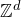by way of long range percolation. In this process, an edge between vertices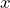and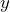is added to the existing graph structure with probability asymptotic to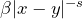, independently of other edges, for some positive parameters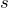and~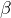. Earlier work identified five different regimes of the asymptotic scaling of the graph-theoretical distance with the Euclidean distance depending on the relative position of the exponentto  numbers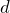and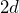. In particular, the graph-theoretical distance scales sublinearly with the Euclidean distance for all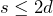and remains bounded for all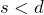.> >    After an overview of known results, I will focus mainly on the regime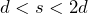. Here the graph theoretical distance between vertices at Euclidean distance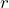is asymptotic to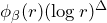as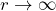, where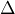is a known function of~and~and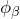is a positive and bounded function subject to the log-log-periodic restriction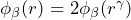for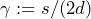. Whileappears to arise from the method of proof and its reliance on subadditivity along doubly-exponential sequences of scales, I will show that it is actually non-constant, at least for~sufficiently large. The resulting arithmetic oscillations (on top of the polylogarithmic growth) are caused by the shortest paths having a dyadic hierarchical structure all the way up to unit scales.> >    The talk is based on a sequence of papers involving, in recent collaborations, Jeff Lin and Andrew Krieger. >

______________________

Based on

https://arxiv.org/abs/2203.17207

http://arxiv.org/abs/1705.10380

## Video